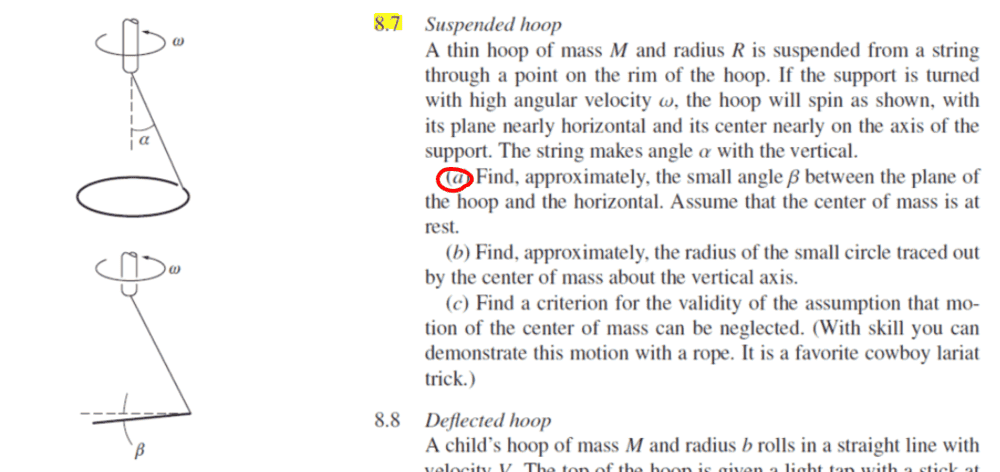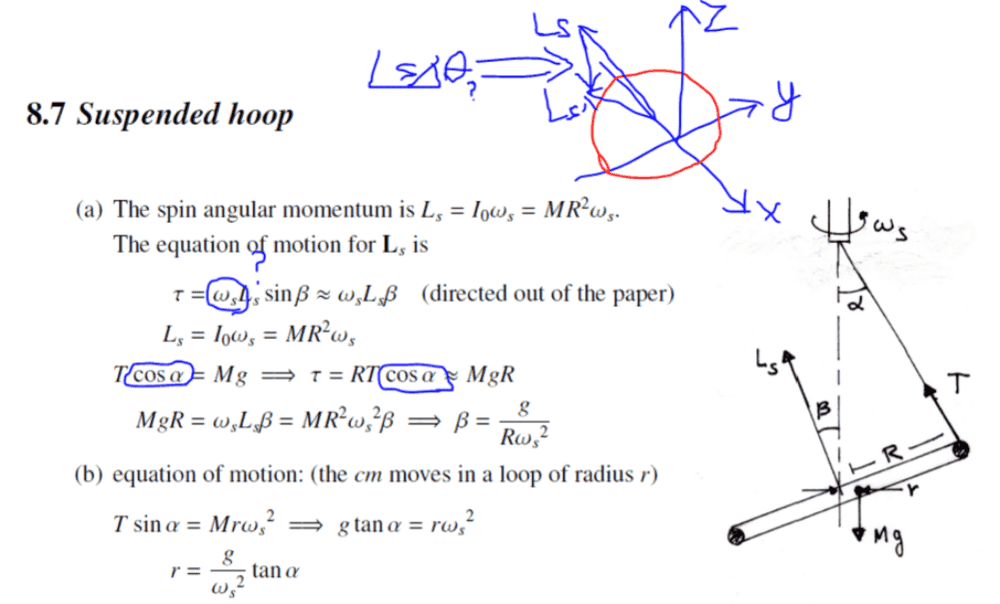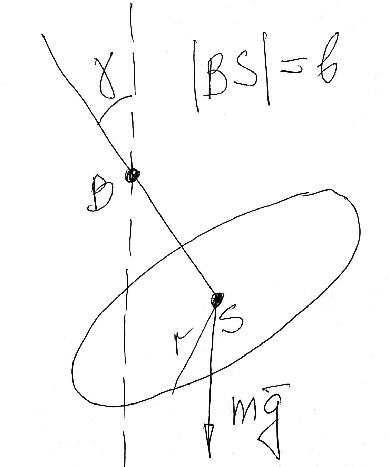# A rotating suspended hoop

Leo Liu
Homework Statement:
A thin hoop of mass M and radius R is suspended from a string through a point on the rim of the hoop. If the support is turned with high angular velocity ω, the hoop will spin as shown, with its plane nearly horizontal and its center nearly on the axis of the support. The string makes angle α with the vertical. (a) Find, approximately, the small angle β between the plane of the hoop and the horizontal. Assume that the center of mass is at rest.
Relevant Equations:
.
Problem (a only):Solution:My questions:
1. The official solution gives no information about the point at which the torque is measured. I thought it was the CM of the hoop, but the torque should be ##\tau=RT\cos(\alpha-\beta)##; the angle was given by a geometric proof. I would like to know where the torque is calculated in the solution.
2. Why is the rate of change of momentum ##L_s\omega_s## rather than ##L_s\dot\theta_y## (see the diagram in blue & red), and why is this term multiplied by ##\sin\beta##? Does this have something to do with the choice of coordinate?

Thank you.

#### Attachments

•Delta2

Homework Helper
Gold Member
2022 Award
1. the torque should be ##\tau=RT\cos(\alpha-\beta)##;
We are given that beta is small, so ##\cos(\alpha-\beta)## is not much different from ##\cos(\alpha)##.

Edit: but see post #11.
2. Why is the rate of change of momentum ##L_s\omega_s## rather than ##L_s\dot\theta_y## (see the diagram in blue & red), and why is this term multiplied by ##\sin\beta##?
Consider Ls as the sum of a constant vertical component and a horizontal component. The horizontal component rotates at rate ##\omega_s## and has magnitude ##L_s\sin\beta##.

Last edited:
•etotheipi and Leo Liu
I believe that for a rigour solution one should first write down exact equations and then take into account that ##\omega## is big (relative what?).
Let ##S## be the center of mass of the ring. Then we have
$$J_S\boldsymbol{\dot\omega}+\boldsymbol\omega\times J_S\boldsymbol\omega=\boldsymbol{SA}\times\boldsymbol T.$$
Here ##\boldsymbol T## is the tension force of the string; ##A## is the point where the string is attached to the ring. It is clear ##\boldsymbol{\dot\omega}=0,\quad \boldsymbol\omega\parallel\boldsymbol g##. We need also ##m\boldsymbol a_S=\boldsymbol T+m\boldsymbol g.##
All these equations should be expanded in a ring-fixed coordinate frame. It is convenient to take a frame ##Sxyz## such that the string belongs to the plane ##Sxz## and ##Sxy## contains the ring.
It is also helpful to exclude the tension first:
$$\boldsymbol\omega\times J_S\boldsymbol\omega=\boldsymbol{SA}\times(m\boldsymbol a_S-m\boldsymbol g).$$

Last edited:
And also, in the gyroscope approximation, ##\mathbf{L} = I_s \omega_s \boldsymbol{e}_1## (##\boldsymbol{e}_1## is a ring-fixed basis vector), so ##d\mathbf{L}/dt = I_s \omega_s (d\mathbf{e}_1 /dt)##, since in this approximation ##\omega_s## is constant. But, ##(d\mathbf{e}_1 /dt) = \boldsymbol{\Omega} \times \boldsymbol{e}_1 = \Omega \sin{(\beta)} \boldsymbol{e}_{\theta}##, so$$d\mathbf{L}/dt = I_s \omega_s \Omega \sin{(\beta)} \boldsymbol{e}_{\theta} = L_s \Omega \sin{(\beta)} \boldsymbol{e}_{\theta}$$where ##\boldsymbol{\Omega}## is the precession angular velocity, and ##\boldsymbol{e}_{\theta}## is a unit vector pointing, in this case, out of the page. That's a general-ish result, that the rate of change of momentum in the gyroscope approximation is roughly of magnitude ##L_s \Omega \sin{\beta}##, if ##\beta## is the angle between ##\boldsymbol{L}## and the precession axis.

(Edited: see below discussion, the gyroscope approximation doesn't seem to be valid here, considering that the spin angular speed is zero and precession angular speed is ##\omega_s##, whilst the gyroscope approximation requires the spin angular speed to greatly exceed the precession angular speed!)

Last edited by a moderator:
•Leo Liu and Delta2
I have got the following
$$\omega^2\Big(\frac{r}{4}\sin(2\gamma)-b\sin^2\gamma\Big)=g\cos\gamma$$

Dotted line is the axis of rotationLast edited:
•etotheipi
Leo Liu
But, ##(d\mathbf{e}_1 /dt) = \boldsymbol{\Omega} \times \boldsymbol{e}_1 = \Omega \sin{(\beta)} \boldsymbol{e}_{\theta}##, so$$d\mathbf{L}/dt = I_s \omega_s \Omega \sin{(\beta)} \boldsymbol{e}_{\theta} = L_s \Omega \sin{(\beta)} \boldsymbol{e}_{\theta}$$where ##\boldsymbol{\Omega}## is the precession angular velocity, and ##\boldsymbol{e}_{\theta}## is a unit vector pointing, in this case, out of the page.
Could you tell me what the direction of ##\hat e_1## is? It seems that it is parallel to the vertical auxiliary line. Would you tell me what the equation will look like if we set our frame in a way that makes ##\hat e_1\parallel \vec\omega_s##?

Could you tell me what the direction of ##\hat e_1## is? It seems that it is parallel to the vertical auxiliary line. Would you tell me what the equation will look like if we set our frame in a way that makes ##\hat e_1\parallel \vec\omega_s##?

The unit vector ##\boldsymbol{e}_1## I used is perpendicular to the plane of the hoop [it is fixed in the hoop-fixed frame], and at angle ##\beta## to the vertical auxiliary line, i.e. in fact indeed ## \boldsymbol{e}_1\parallel \boldsymbol{\omega}_s##. And this unit vector is rotating around the vertical auxiliary line, i.e.

$$\frac{d\boldsymbol{e}_1}{dt} = \boldsymbol{\Omega} \times \boldsymbol{e}_1$$

•Leo Liu
Leo Liu
The unit vector ##\boldsymbol{e}_1## I used is perpendicular to the plane of the hoop [it is fixed in the hoop-fixed frame], and at angle ##\beta## to the vertical auxiliary line, i.e. in fact indeed ## \boldsymbol{e}_1\parallel \boldsymbol{\omega}_s##. And this unit vector is rotating around the vertical auxiliary line, i.e.

$$\frac{d\boldsymbol{e}_1}{dt} = \boldsymbol{\Omega} \times \boldsymbol{e}_1$$
Thanks--now I understand what you are trying to do. But can you please explain why the expression ##\Delta\tau=L_s\Delta\theta_y## doesn't work, if we consider that the change in the direction of the angular momentum ##L_s## is caused by the rotation of the hoop around the y-axis (see the diagram I drew)?

Edit: Just found that the origin of the frame to which ##\dot{\vec r}=\vec\omega\times\vec r## is applicable has to be on the axis of rotation. So I guess that's why both you and the solution try to convert ##L_s## into a perpendicular angular momentum vector rotating about the auxiliary line about which ##L_s## precesses.

Last edited:
I feel that I do not understand how to derive approximate formulas which are proposed in the initial post from the exact formulas. I have a doubt regarding correctness of the problem statement.

Last edited:
••Delta2 and etotheipi
Homework Helper
Gold Member
2022 Award
The principle behind the book's analysis is that we can think of the motion of the hoop as a rotation at rate ω about an axis at angle β to the vertical (angular momentum L), but with the axis precessing about a vertical axis at the same rate ω.
Is this valid? Seems reasonable ...

Vertical balance:
##T\cos(\alpha)=Mg##
Precession:
##L=I\omega##
##\tau=\omega L\sin(\beta)=I\omega^2\sin(\beta)##
Torque balance:
##\tau=TR\cos(\alpha-\beta)##

I often see approximations made prematurely, so let's avoid them for now.
##\tau=TR(\cos(\alpha)cos(\beta)+\sin(\alpha)\sin(\beta))##
##=Mg\sec(\alpha)R(\cos(\alpha)cos(\beta)+\sin(\alpha)\sin(\beta))##
Hence
##MR^2\omega^2\sin(\beta)=Mg\sec(\alpha)R(\cos(\alpha)cos(\beta)+\sin(\alpha)\sin(\beta))##
##R\omega^2\sin(\beta)=g(cos(\beta)+\tan(\alpha)\sin(\beta))##
##\tan(\beta)=\frac g{R\omega^2-g\tan(\alpha)}##.

No approximations made, so should be right in all cases. But consider the case ##\alpha=0##. We should get ##\beta=\pi/2##. Doesn't work.
Indeed, in this extreme case L=0.

•etotheipi and Delta2
Homework Helper
Gold Member
2022 Award
I now think the best way to approach this is in the frame of reference of the rotating vertical axis. This gives a static arrangement, with a non-uniform centrifugal force on the hoop. That supplies a torque to balance the weight of the hoop.

As a simplified example, consider a rod length 2L instead of a hoop, and its mass centre is further from the axis than is the point of attachment. The string has length S.
##M=2L\rho##
##T\cos(\alpha)=Mg##
##T\sin(\alpha)=M\omega^2(S\sin(\alpha)+L\cos(\beta))##
If x is the distance along the rod from the attachment point, the centrifugal force on an element dx is ##\omega^2(S\sin(\alpha)+x\cos(\beta))\rho.dx##.
The torque balance on the rod about its point of attachment gives
##MgL\cos(\beta))=\int_0^{2L}\omega^2(S\sin(\alpha)+x\cos(\beta))x\sin(\beta)\rho.dx##.

Figuring out the net torque from the centrifugal force on the tilted and off-centre hoop will be trickier.

The principle behind the book's analysis is that we can think of the motion of the hoop as a rotation at rate ω about an axis at angle β to the vertical (angular momentum L), but with the axis precessing about a vertical axis at the same rate ω.
I assume that the system moves such that the hoop and the string form a rigid body. The string is at rest relative the hoop. And this rigid body rotates with constant angular velocity ##\boldsymbol\omega##. The vector ##\boldsymbol\omega## is directed vertically. Is this the same ?

•etotheipi
Homework Helper
Gold Member
2022 Award
I assume that the system moves such that the hoop and the string form a rigid body. The string is at rest relative the hoop. And this rigid body rotates with constant angular velocity ##\boldsymbol\omega##. The vector ##\boldsymbol\omega## is directed vertically. Is this the same ?
Yes. But I concluded it cannot be a valid view.
OTOH, I realize now my analysis in post #10 assumed the mass centre is directly below the ceiling support, which will not be so in general.

•Leo Liu and etotheipi
Yes. But I concluded it cannot be a valid view.
what do you mean?

I think, the book goes wrong by treating this as a precession problem (and using the gyroscopic approximation), whilst this would require that the precession speed about the vertical axis is much smaller than the spin speed about the disks own axis.

But in fact the spin angular speed of the disk about its own axis is zero, whilst the rotation speed of the configuration about the vertical axis is ##\omega_s##. So the equation ##\tau = L_s \omega_s \sin{\beta}## is completely unjustified here.

•wrobel
Homework Helper
Gold Member
2022 Award
what do you mean?
As I noted in post #10, it does not give the right result when α is zero and the hoop hangs vertically, i.e.with its axis rotating in the horizontal plane.

As I noted in post #10, it does not give the right result
Actually it does give. In the motion you are speaking about, the angular velocity can be whatever you want . Equality from #5 takes the form 0=0

Last edited:
Homework Helper
Gold Member
2022 Award
Actually it does give. In the motion you are speaking about, the angular velocity can be whatever you want . Equality from #5 takes the form 0=0
But to get the equation in #5 you did not use the approach I described in post #10, did you?

But to get the equation in #5 you did not use the approach I described in post #10, did you?
I used the general equations of motion for the rigid body (#3)

Homework Helper
Gold Member
2022 Award
I used the general equations of motion for the rigid body (#3)
Right. In post #13 I meant that the book approach I described in post #10 cannot be valid.

Homework Helper
Gold Member
2022 Award
I have got the following
$$\omega^2\Big(\frac{r}{4}\sin(2\gamma)-b\sin^2\gamma\Big)=g\cos\gamma$$
To a first approximation that gives ##\gamma=\frac{2g}{\omega^2R}##, double the book value, right?

yes, that is right, provided we consider the distance ##\delta =b\sin\gamma## between the center of the hoop and the axis of rotation to be small relative r

Last edited:
Leo Liu
I now think the best way to approach this is in the frame of reference of the rotating vertical axis. This gives a static arrangement, with a non-uniform centrifugal force on the hoop. That supplies a torque to balance the weight of the hoop.
I wonder if it is possible to apply Euler's equations of rotation to this problem. I have tried it, but it didn't seem to work.
(Edited) Assume axes 123 are placed on the hoop in a way that the hoop is symmetrical about all of them.
$$\tau_1=\dot L_1=I_s\dot\omega_s+\underbrace{(MR^2-\frac 1 2 MR^2)}_{I_3-I_2}\omega_s\omega_2=RT\cos\alpha$$

Last edited:
Homework Helper
Gold Member
I wonder if it is possible to apply Euler's equations of rotation to this problem. I have tried it, but it didn't seem to work.
Assume axes 123 are placed on the hoop in a way that the hoop is symmetrical about all of them.
$$\tau_1=\dot L_1=I_s\dot\omega_s+\underbrace{(I_3-I_2)}_{=0}\omega_3\omega_2=0\neq RT\cos\alpha$$

Euler's equation for the "1" component is $$\tau_1=I_1\dot\omega_1+(I_3-I_2)\omega_3\omega_2$$
The torque due to the tension in the string is out of the page at the instant shown in the figure. So, choose "1" to be out of the page. The "2" axis can be chosen in the plane of the loop in the direction from the center of the loop to the point of attachment of the string. Then "3" is perpendicular to the plane of the loop. Thus, ##I_3 \neq I_2##.

•Leo Liu
I wonder if it is possible to apply Euler's equations of rotation to this problem.
For the Euler equations, we can do what @wrobel did, and hopefully end up with the same answer! In the ring-fixed frame let ##\mathbf{e}_3## be orthogonal to the plane of the ring, and ##\mathbf{e}_1## and ##\mathbf{e}_2## be in the plane of the ring, such that the set is right handed and has ##\mathbf{e}_1## pointing from the centre of the ring to the point where the string meets the ring. Then the inertia tensor written in the basis is$$I = mr^2 \mathbf{e}_3 \otimes \mathbf{e}_3 + \frac{1}{2}mr^2 (\mathbf{e}_1 \otimes \mathbf{e}_1 + \mathbf{e}_2 \otimes \mathbf{e}_2)$$by the perpendicular axis theorem. In this frame the angular velocity of the body is$$\boldsymbol{\omega} = \omega \sin{\beta} \mathbf{e}_1 + \omega \cos{\beta} \mathbf{e}_3$$Whilst the torque is$$\boldsymbol{\tau} = -rT\cos{(\alpha - \beta)} \mathbf{e}_2$$From the force equation ##\mathbf{T} + m\mathbf{g} = m\ddot{\mathbf{x}}_{\text{CM}}##, we obtain the following equations$$T\cos{\alpha} = mg, \quad T\sin{\alpha} = m(l\sin{\beta})\omega^2$$where ##l## is the distance from the hinge to the centre of the ring. On division this yields that ##\tan{\alpha} = (l \omega^2 \sin{\beta})/g##. Applying one of the Euler equations gives$$\tau_2 = I_2 \dot{\omega_2} + (I_1 - I_3) \omega_1 \omega_3 = 0 - \frac{1}{2}mr^2 \omega^2 \sin{\beta} \cos{\beta} = - \frac{1}{4}mr^2 \omega^2 \sin{2\beta}$$Since the ##\tau_2## can be simplified using trigonometry formulae, as well as the Newton II equations, to\begin{align*} \tau_2 &= -\frac{mgr}{\cos{\alpha}} \left( \cos{\alpha} \cos{\beta} + \sin{\alpha} \sin{\beta} \right) \\ &= - mgr\cos{\beta} - mgr \tan{\alpha} \sin{\beta} \end{align*}we finally end up with$$g\cos{\beta} + g \tan{\alpha} \sin{\beta} = \frac{1}{4}r \omega^2 \sin{2\beta}$$and substituting in our result for ##\tan{\alpha}##,$$g\cos{\beta} = \frac{1}{4}r \omega^2 \sin{2\beta} - l\omega^2 \sin^2{\beta} = \omega^2 \left( \frac{1}{4}r \sin{2\beta} - l \sin^2{\beta} \right)$$Then like @haruspex pointed out, if you as a first approximation take ##\sin{\beta} \rightarrow \beta##, ##\cos{\beta} \rightarrow 1##, and ##\beta^2 \rightarrow 0##, you get ##\beta \approx (2g)/(r\omega^2)##

•Leo Liu
•